# Math Formulas You Must Know and Understand

What do you need in order to excel at anything? A mastery of the fundamentals. This is true for master athletes, master artists, and great musicians. It’s also true for those who want to master QUANT math.

In the math of the QUANT, certain formulas are your fundamentals. There are five QUANT formulas that you should study and fully understand. (Simply memorizing a formula is never enough, and memorizing a formula without truly understanding it can actually hurt you on the exam!)

These five QUANT formulas are five powerful tools to fuel your success on QUANT Math. Find out how much each can help you! Below, I’ve given you a breakdown of each formula, followed by practice questions so that you can test yourself on how well you understand these key equations. Try each one and then check the answers and explanations.

## 1. The Pythagorean Theorem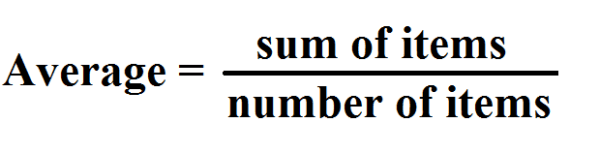You probably already know the Pythagorean Theorem, even if you think you don’t. You no doubt studied this formula in middle school and high school math, and possibly in undergraduate math classes too. Pythagoras’s theorem is somewhere in the back of your mind. Bering it to the surface for your QUANT Quant prep!

The Pythagorean Theorem applies to right triangles. A right triangle is any triangle where one angle is a right angle, an angle that measures 90 degrees. This theorem states that for any right triangle with sides a and b on the right angle, and side c opposite the right angle, a^2 + b^2 = c^2.

The a^2 + b^2 = c^2 formula is a powerful tool for solving many different QUANT Quant geometry questions.

Here’s an example:

In the coordinate plane, point A has coordinates (-2, -1) and point B has coordinates (4, 7). What is the length of segment AB?

(A) 10

(B) 10.5

(C) 12

(D) 13.675

(E) 14

### Explanation

For an answer and explanation to the problem above, see our post on the Pythagorean Theorem. This post also contains additional practice questions, and additional help with this very valuable QUANT math formula.

## 2. The formula for the area of a triangle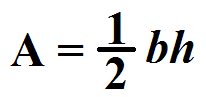This triangle formula is even more basic than the one from Pythagoras. The area of a triangle can be calculated by remembering that A (for area) is equal to one half of the base of the triangle multiplied by the height. Written in plain text, this formula is expressed as:

A = \dfrac{1}{2}bh

Again, we have a seemingly simple formula that can allow you to solve all kinds of complex math problems on the QUANT.

Here’s one sample question that uses A = \dfrac{1}{2}bh: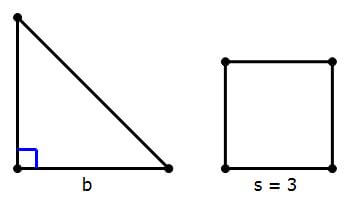The figure on the left is an isosceles right triangle, and the figure on the right is a square of length 3. Find the value of b.

Statement #1: b is the length of the diagonal of the square.

Statement #2: the triangle and the square have the same area.

(A) Statement 1 alone is sufficient but statement 2 alone is not sufficient to answer the question asked.

(B) Statement 2 alone is sufficient but statement 1 alone is not sufficient to answer the question asked.

(C) Both statements 1 and 2 together are sufficient to answer the question but neither statement is sufficient alone.

(D) Each statement alone is sufficient to answer the question.

### Explanation

The answer and explanation for the question above is found in the "Area of a Triangle". This post can help you really master this formula, so that you truly understand how the formula works. (Again, don’t just memorize the formula itself.)

## 3. The formulas for averages and sums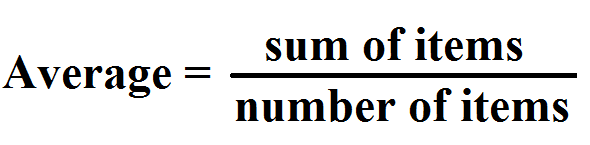And here we have yet another middle school formula that you can now use on the QUANT. (When your teachers told you that you really would use all of that math again someday, they weren’t kidding!)

Actually, here we have not one formula, but a have a pair of closely related formulas: the formula for the average value of a set of numbers, and the formula for the total sum of a set of numbers.

To get the average of a numerical set, use this formula:

average = \dfrac{(sum \space of \space items)}{(number \space of \space items)}

Now, suppose a QUANT problem gives the average, but you need to find the sum. This is where the formula for the sum comes in handy:

sum \space of \space items = (average)*(number \space of \space items)

Here is a typical QUANT math question involving averages and sums:

There are 17 students in a certain class. On the day the test was given, Taqeesha was absent. The other 16 students took the test, and their average was 77. The next day, Taqeesha took the test, and with her grade included, the new average is 78. What is Taqeesha’s grade on the test?

(A) 78

(B) 80

(C) 87

(D) 91

(E) 94

### Explanation

As usual, we have a full explanation and some extra help with the formulas… in a post just for this concept. Check out our article on Averages and Sums Formulas.

## 4.The distance/rate/time and work/rate/time formulas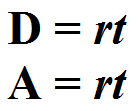On the QUANT, you’ll see story problems about the rate of speed that someone is travelling. And you’ll see story problems about how quickly a person or machine completes a certain kind of work. As you might expect, rate of speed and rate of productivity are very similar mathematical concepts. And these two types of calculations have similar formulas.

Distance traveled is equal to rate of speed multiplied by time spent travelling or moving. The formula for this is d = rt. (Sometimes this is called “the dirt formula.”)

Similarly, amount worked is equal to the rate of productivity multiplied by the time spent working. This work rate formula can be expressed as a = rt. (Nickname: “the art formula.”)

Both of these formulas suggest a number of other relationships between distance, amount, rate, and time. For instance, if d = rt, and a = rt, then r must equal \dfrac{d}{t}, and it follows that r = \dfrac{a}{t} in the work rate formula. There are a number of other ways you can play with these two similar formulas in QUANT math operations.

Let’s look at a couple of sample QUANT math problems where you need to know and understand the concepts behind the “dirt” and “art” equations:

1) A car drives 40 miles on local roads at 20 mph, and 180 miles on the highway at 60 mph, what is the average speed of the entire trip?

(A) 36 mph

(B) 40 mph

(C) 44 mph

(D) 52 mph

(E) 58 mph

2) When Mary paints a house, it takes her 4 hours. When Lisa joins Mary, and they work together, it takes them only 3 hours to paint a house of the same size. How long would it take for Lisa to paint a house of the same size by herself?

(A) 5 hr

(B) 6 hr

(C) 7 hr

(D) 12 hr

(E) 20 hr

### Explanation

For answers and explanations to these two questions, go to "Distance and Work Rate" tutorial. As always, you get some extra tips and tricks with your explanations.

## 5. The permutation and combination formulas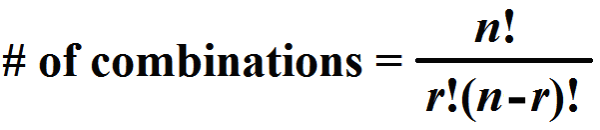This is the one truly complicated pair of formulas among our list of “must have” formulas for the QUANT. It’s unlikely that you encountered the equations for permutations or combinations in junior high. And you may have only touched on these formulas in high school.

To understand these formulas, you need to first be familiar with the use of the exclamation point (!) as a mathematical notation. In QUANT Quant, when you see a number followed by an exclamation point, it means you are supposed to multiply that number by all positive whole numbers that are smaller than it.

So, 4! = 4*3*2*1. 11! = 11*10*9*8*7*6*5*4*3*2*1. 1! would merely equal 1, because there are no smaller positive whole numbers of less than 1. And so on. You get the idea.

This brings us to permutation. A permutation is the total possible ways that you can arrange a set of things in different order. When a set of things is small, permutations are simple. If you have, for instance, book A and book B, then you can arrange those books in 2 ways: AB or BA. Now, suppose you have ball A, ball B, and ball C. In that case, you can arrange the objects in your set in 6 ways: ABC, ACB, CAB, CBA, BCA, and BAC.

Both of these permutations can be expressed by using the “!” notation. Take the number of objects and apply the notation. For a set containing only A and B, there are 2 objects. 2! = 2*1 = 2, which means there are two possible arrangements. Then, for the set containing three objects, A, B, and C, 3! = 3*2*1 = 6 possible arrangements.

So the permutation formula is simply:

permutation = n!

A combination is a group of things taken from a larger set. Combinations are more complicated than permutations in some ways. With combinations, the order that the objects are arranged doesn’t matter. AB and BA are two permutations, but they are not two combinations. This is because both AB and BA have the exact same two things in them: item A and item B.

For combination projects on the QUANT, you’ll be given the number of things in a set, or n. Then you’ll be given the number of things that will go into combinations taken from the set, or r. So if you are asked how many combinations of 3 things can be taken from a set of 20 things, n = 20, and r = 3.

With that in mind, the formula for combinations is:

\# of \space combinations = \dfrac{n!}{r!(n-r)!}

This formula can look tricky, but remember–you’re only dealing with two variables, n and r. Understand what n and r mean, and you’ll be able to master this essential QUANT math formula for combinations.

OK, now for some example QUANT Quant problems that use this duo of essential QUANT formulas:

1) A bookseller has two display windows. She plans to display 4 new fiction books in the left window, and 3 new non-fiction books in the right window. Assuming she can put the four fiction books in any order, and separately, the three non-fiction books in any order, how many total configurations will there be for the two display windows?

(A) 24

(B) 72

(C) 144

(D) 336

(E) 420

2) The county-mandated guidelines at a certain community college specify that for the introductory English class, the professor may choose one of three specified novels, and choose two from a list of 5 specified plays. Thus, the reading list for this introductory class is guaranteed to have one novel and two plays. How many different reading lists could a professor create within these parameters?

(A) 15

(B) 30

(C) 90

(D) 150

(E) 360

### Explanations

You guessed it! The explanations for these questions appear in a special page Permutations and Combinations.